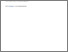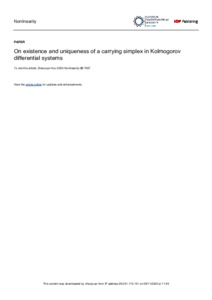# On existence and uniqueness of a carrying simplex in Kolmogorov differential systems

Hou, Zhanyuan (2020) On existence and uniqueness of a carrying simplex in Kolmogorov differential systems. Nonlinearity, 33 (12). ISSN 0951-7715Preview
Text
Hou_On-existence.pdf - Accepted Version

Official URL: https://doi.org/10.1088/1361-6544/abb03c

## Abstract / Description

This paper deals with global asymptotic behaviour of the dynamics for $N$-dimensional competitive Kolmogorov differential systems of equations $\frac{dx_i}{dt} =x_if_i(x), 1\leq i\leq N, x\in \R^N_+$. A theory based on monotone dynamical systems was well established by Morris W Hirsch (Systems of differential equations which are competitive or cooperative: III. Competing species, Nonlinearity, 1 (1988), 51--71). One of his theorems is outstanding and states the existence of a co-dimension 1 compact invariant submanifold $\Sigma$ that attracts all the nontrivial orbits under certain assumptions and, in practice, under the condition that the system is totally competitive (all $N^2$ entries of the Jacobian matrix $Df$ are negative). The submanifold $\Sigma$ has been called carrying simplex since then and the theorem has been well accepted with many hundreds of citations. In this paper, we point out that the requirement of total competition is too restrictive and too strong; we prove the existence and uniqueness of a carry simplex under the assumption of strong internal competition only (i.e. $N$ diagonal entries of $Df$ are negative), a much weaker condition than total competition. Thus, we improve the theorem significantly by dramatic cost reduction from requiring $N^2$ to $N$ negative entries of $Df$. As an example of applications of the main result, the existence and global attraction (repulsion) of a heteroclinic limit cycle for three-dimensional systems is discussed and two concrete examples are given to demonstrate the existence of such heteroclinic cycles.

Item Type: Article competitive Kolmogorov systems; carrying simplex, existence and uniqueness; heteroclinic limit cycles; global attraction; global repulsion 500 Natural Sciences and Mathematics > 510 Mathematics500 Natural Sciences and Mathematics > 570 Life sciences; biology School of Computing and Digital Media Zhanyuan Hou 20 Aug 2020 08:20 09 Nov 2021 01:58 https://repository.londonmet.ac.uk/id/eprint/6000View Item